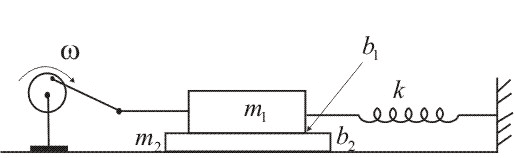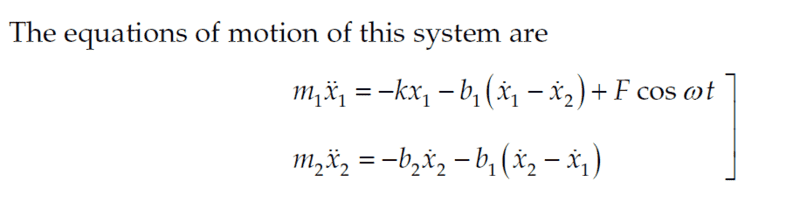ANAli
Homework Statement:
(Problem 3.26; Classical Dynamics of Particles and Systems)
Figure 3-B illustrates a mass m1 driven by a sinusoidal force whose frequency is w. The mass m1 is attached to a rigid support by a spring of force constant k and slides on a second mass m2. The frictional force between m1 and m2 is represented by the damping parameter b1 and the friction force between m2 and the support is represented by b2. Construct the electrical analog of the system, and calculate the impedance.
Relevant Equations:
Inserted image of equations in question. These are from the solution manual.I am having trouble understanding why the second term in the m2 equation, b1(x'2 - x'1), is a negative term. Given that this force is the reason why m2 is moving in the first place, why is it not considered a driving force? I think that I don't have a clear understanding of what driving force means.

Last edited by a moderator:

Staff Emeritus
Homework Helper
Gold Member
A driving force is an external force imposed on the system that drives its motion. The frictional force is not driving because it is an internal force between different parts of your system that is not forcing the motion of the system but instead its form depends on the motion of the system.

Since it is the frictional force from 1 on 2, it is the 3rd law pair of the frictional force from 2 on 1. It must be equal in magnitude and opposite in direction.

•Lnewqban
Homework Helper
Gold Member
What happens if the value of b1 is so big that v1=v2?

•ANAli
ANAli
A driving force is an external force imposed on the system that drives its motion. The frictional force is not driving because it is an internal force between different parts of your system that is not forcing the motion of the system but instead its form depends on the motion of the system.

Since it is the frictional force from 1 on 2, it is the 3rd law pair of the frictional force from 2 on 1. It must be equal in magnitude and opposite in direction.
Got it. I understand. Thank you!

•berkeman
Staff Emeritus